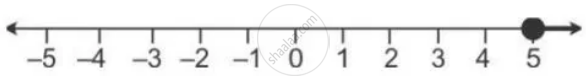Share

# If P = {X: 7x — 4 > 5x + 2, X ∈ R} and Q = {X: X — 19 ≥ 1 — 3x, X ∈ R}, Find the Range of Set P ∩ Q and Represent It on a Number Line. - Mathematics

Course

#### Question

If P = {x: 7x — 4 > 5x + 2, x ∈ R} and Q = {x: x — 19 ≥ 1 — 3x, x ∈ R}, find the range of set P ∩ Q and represent it on a number line.

#### Solution

P = {x : 7x - 4 > 5x + 2, x ∈ R}

7x - 4 > 5x + 2

7x - 5x > 2 + 4

2x > 6

x > 3

Q = {x : x - 19 >= 1 - 3x, x ∈  R}

x - 19 >= 1 - 3x

x + 3x >= 1 + 19

4x  >= 20

x >= 5

P ∩ Q = {x : x >= 5, x ∈ R}Is there an error in this question or solution?

#### APPEARS IN

Selina Solution for Concise Mathematics for Class 10 ICSE (2020 (Latest))
Chapter 4: Linear Inequations (In one variable)
Exercise 4(B) | Q: 19 | Page no. 50
Solution If P = {X: 7x — 4 > 5x + 2, X ∈ R} and Q = {X: X — 19 ≥ 1 — 3x, X ∈ R}, Find the Range of Set P ∩ Q and Represent It on a Number Line. Concept: Representation of Solution on the Number Line.
S